Auxiliary Materials for: A Local Cellular Model for Growth on Quasicrystals

by Prince Chidyagwai and Cliff Reiter, August 2004

The Local Model

We consider growth models on quasicrystaline structures produced via canonical projection. Each cell (vertex) contains a real value with the following process for updating cells. We consider a cell to be solid if its value is greater than or equal to one. Then classify each cell at each step as either receptive or nonreceptive. Cells which are solid and their immediate neighbors are receptive. The updated value is the sum of two terms. The first term is the value of the receptive sites with a constant γ added; the second term is the average of the nonreceptive sites (with receptive sites contributing values of zero to the averages). The averaging consists of taking the average of the centervalue and the average of the immediate neighbors (with zeros as noted above). We usually begin with a single seed of value one at the center of symmetry of the quasicrystalline structure with all other sites initialized at some background level β. Boundary conditions maintain the background level at a radius near the edge of the structure.

Some β-γ Tables of the Growth on several structures: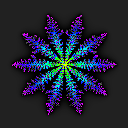Quasicrystaline structure from Z5.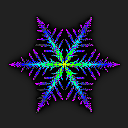Crystaline structure from Z6.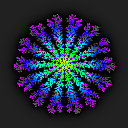Quasicrystaline structure from Z7.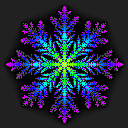Quasicrystaline structure from Z8.

Some β-γ Tables on Variations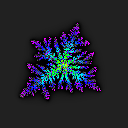Quasicrystaline structure from Z5 on a nonsymmetric initial point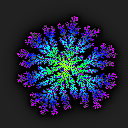Quasicrystaline structure from Z7 on a nonsymmetric initial point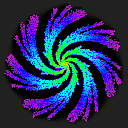Quasicrystaline structure from Z7 with neighbor weights biased by angle

Animations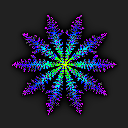Time evolution for β=0.35, γ=0.0001, n = 5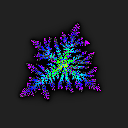Time evolution for β=0.35, γ=0.0001, n = 5 with nonsymmetric initial pointTime evolution for β=0.35, γ=0.0001, n = 7, with cyclic bias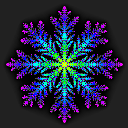Time evolution for β=0.35, γ=0.0001, n = 8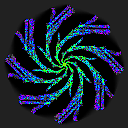Result for β=0.35, γ=0.0001, n = 8 as the bias varies
(if your browser does not display the animations directly, try saving the animation files and then view them)